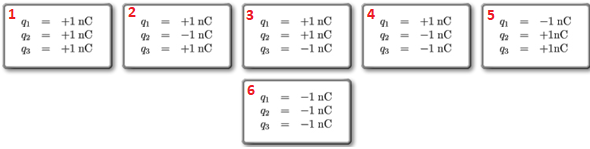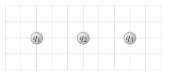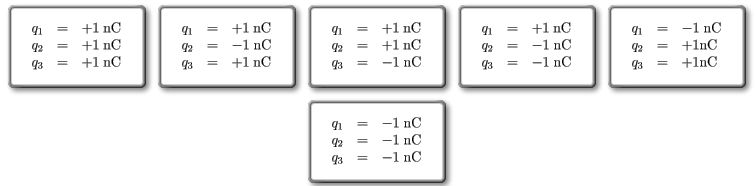# Problem: In the diagram below, there are three collinear point charges: q1, q2, and q3. The distance between q1 and q2 is the same as that between q2 and q3Rank the six combinations of electric charges on the basis of the electric force acting on q1. Define forces pointing to the right as positive and forces pointing to the left as negative. Rank positive forces as larger than negative forces. Rank from largest to smallest, placing the largest on the left and the smallest on the right.

###### FREE Expert Solution

Coulomb's law:

$\overline{){\mathbf{F}}{\mathbf{=}}\frac{{\mathbf{kq}}_{\mathbf{1}}{\mathbf{q}}_{\mathbf{2}}}{{\mathbf{r}}^{\mathbf{2}}}}$1

F1 = F12 + F13 = kq1q2/(r12)2 + kq1q3/(r13)2 = k(1)(1)/r2 + k(1)(1)/(2r)2 = 1.25k/r2

q1 is repelled to the left by the two charges.

F1 = -1.25k/r2

2

F2 = F12 + F13 = kq1q2/(r12)2 + kq1q3/(r13)2 = k(1)(-1)/r2 + k(1)(1)/(2r)2 = -0.75k/r2

q1 is attracted strongly to q2 and repelled less by q3. The net force is towards q2.

81% (231 ratings)###### Problem Details

In the diagram below, there are three collinear point charges: q1, q2, and q3. The distance between q1 and q2 is the same as that between q2 and q3Rank the six combinations of electric charges on the basis of the electric force acting on q1. Define forces pointing to the right as positive and forces pointing to the left as negative. Rank positive forces as larger than negative forces. Rank from largest to smallest, placing the largest on the left and the smallest on the right.